×#### Thank you for registering.

One of our academic counsellors will contact you within 1 working day.

Click to Chat

1800-1023-196

+91-120-4616500

CART 0

• 0

MY CART (5)

Use Coupon: CART20 and get 20% off on all online Study Material

ITEM
DETAILS
MRP
DISCOUNT
FINAL PRICE
Total Price: Rs.

There are no items in this cart.
Continue Shopping• Complete JEE Main/Advanced Course and Test Series
• OFFERED PRICE: Rs. 15,900
• View Details

```Revision Notes on Squares and Square Roots

Square Number

Any natural number ‘p’ which can be represented as y2, where y is a natural number, then ‘p’ is called a Square Number.

Example

4 = 22

9 = 32

16 = 42

Where 2, 3, 4 are the natural numbers and 4, 9, 16 are the respective square numbers.

Such types of numbers are also known as Perfect Squares.

Some of the Square Numbers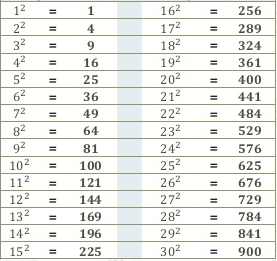Properties of Square Numbers

We can see that the square numbers are ending with 0, 1, 4, 5, 6 or 9 only. None of the square number is ending with 2, 3, 7 or 8.

Any number having 1 or 9 in its one’s place will always have a square ending with 1.

Number
Square Number

1
1

9
81

11
121

19
361

21
441

Any number which has 4 or 6 in its unit’s place, its square will always end with 6.

Number
Square Number

4
16

16
256

24
576

36
1296

44
1936

Any number which has 0 in its unit’s place, its square will always have an even number of zeros at the end.

Number
Square number

10
100

50
2500

100
10000

150
22500

400
160000

Some More Interesting Patterns

If we could arrange the dotted pattern of the numbers in a triangular form then these numbers are called Triangular Numbers. If we add two consecutive triangular numbers then we can get the square number.2. Numbers between Square Numbers

If we take two consecutive numbers n and n + 1, then there will be (2n) non-perfect square numbers between their squares numbers.

Example

Let’s take n = 5 and 52 = 25

n + 1 = 5 + 1 = 6 and 62 = 36

2n = 2(5) = 10

There must be 10 numbers between 25 and 36.

The numbers are 26, 27, 28, 29, 30, 31, 32, 33, 34, 35.

Sum of first n natural odd numbers is n2.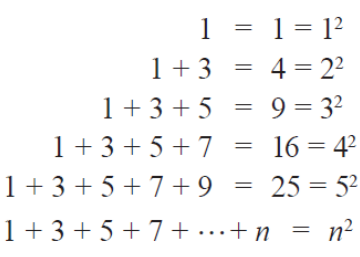Any square number must be the sum of consecutive odd numbers starting from 1.

And if any natural number which is not a sum of successive odd natural numbers starting with 1, then it will not be a perfect square.

4. A Sum of Consecutive Natural Numbers

Every square number is the summation of two consecutive positive natural numbers.

If we are finding the square of n the to find the two consecutive natural numbers we can use the formula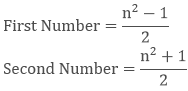Example

52 = 2512 + 13 = 25

Likewise, you can check for other numbers like

112 = 121 = 60 + 61

5. The Product of Two Consecutive Even or Odd Natural Numbers

If we have two consecutive odd or even numbers (a + 1) and (a -1) then their product will be (a2- 1)

Example

Let take two consecutive odd numbers 21 and 23.

21 × 23 = (20 - 1) × (20 + 1) = 202 - 1

6. Some More Interesting Patterns about Square Numbers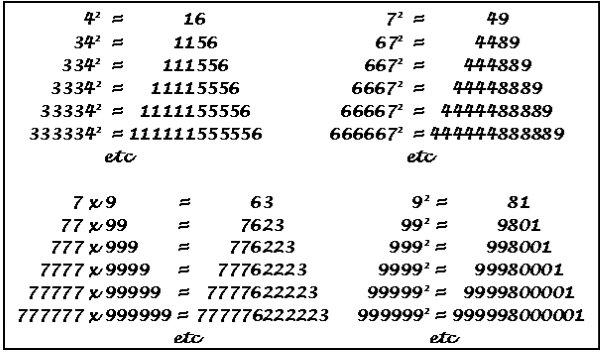Finding the Square of a Number

To find the square of any number we needed to divide the number into two parts then we can solve it easily.

If number is ‘x’ then x = (p + q) and x2 = (p + q)2

You can also use the formula (p + q)2 = p2 + 2pq + q2

Example

Find the square of 53.

Solution:

Divide the number in two parts.

53 = 50 + 3

532 = (50 + 3)2

= (50 + 3) (50 + 3)

= 50(50 + 3) +3(50 + 3)

= 2500 + 150 + 150 + 9

= 2809

1. Other pattern for the number ending with 5

For numbers ending with 5 we can use the pattern

(a5)2 = a × (a + 1)100 + 25

Example

252 = 625 = (2 × 3) 100 + 25

452 = 2025 = (4 × 5) 100 + 25

952 = 9025 = (9 × 10) 100 + 25

1252 = 15625 = (12 × 13) 100 + 25

2. Pythagorean Triplets

If the sum of two square numbers is also a square number, then these three numbers form a Pythagorean triplet.

For any natural number p >1, we have (2p) 2 + (p2 -1)2 = (p2 + 1)2. So, 2p, p2-1 and p2+1 forms a Pythagorean triplet.

Example

Write a Pythagorean triplet having 22 as one its member.

Solution:

Let 2p = 6

P = 3

p2 + 1 = 10

p2 - 1 = 8.

Thus, the Pythagorean triplet is 6, 8 and 10.

62 + 82 = 102

36 + 64 = 100

Square Roots

The square root is the inverse operation of squaring. To find the number with the given square is called the Square Root.

22 = 4, so the square root of 4 is 2

102 = 100, therefore square root of 100 is 10

There are two square roots of any number. One is positive and other is negative.

The square root of 100 could be 10 or -10.

Symbol of Positive Square Root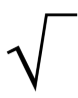Finding Square Root

1. Through Repeated Subtraction

As we know that every square number is the sum of consecutive odd natural numbers starting from 1, so we can find the square root by doing opposite because root is the inverse of the square.

We need to subtract the odd natural numbers starting from 1 from the given square number until the remainder is zero to get its square root.

The number of steps will be the square root of that square number.

Example

Calculate the square root of 64 by repeated addition.

Solution:

64 – 1 = 63

63 – 3 = 60

60 – 5 = 55

55 – 7 = 48

28 – 13 = 15

48 – 9 = 39

15 – 15 = 0

39 – 11 = 28

2. Prime Factorization

In this method, we need to list the prime factors of the given number and then make the pair of two same numbers.

Then write one number for each pair and multiply to find the square root.

Example

Calculate the square root of 784 using prime factorization method.

Solution:

List the prime factors of 784.

784 = 2 × 2 × 2 × 2 × 7 × 7

√784 = 2 × 2 × 7 = 28

3. Division Method

Steps to find the square root by division method

Step 1: First we have to start making the pair of digits starting from the right and if there are odd number of digits then the single digit left over at the left will also have bar .

Step 2: Take the largest possible number whose square is less than or equal to the number which is on the first bar from the left. Write the same number as the divisor and the quotient with the number under the extreme left bar as the dividend. Divide to get the remainder.

Step 3: Like a normal division process bring the digits in next bar down and write next to the remainder.

Step 4: In next part the quotient will get double and we will right in next line with a blank on its right.

Step 5: Now we have to take a number to fill the blank so that the if we take it as quotient then the product of the new divisor and the new digit in quotient is less than or equal to the dividend.

Step 6: If there are large number of digits then you can repeat the steps 3, 4, 5 until the remainder does not become 0.

Example

Calculate the square root of √729 using division method.

Solution: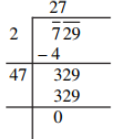Thus, √729 = 27.

Square Roots of Decimals

To find the square root of a decimal number we have to put bars on the primary part of the number in the same manner as we did above. And for the digits on the right of the decimal we have to put bars starting from the first decimal place.
Rest of the method is same as above. We just need to put the decimal in between when the decimal will come in the division.

Example

Find √7.29 using division method.

Solution: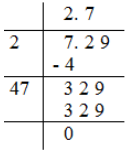Thus, √7.29 = 2.7

Remark: To put the bar on a number like 174.241, we will put a bar on 74 and a bar on 1 as it is a single digit left. And in the numbers after decimal, we will put a bar on 24 and put zero after 1 to make it double-digit.

174. 24 10

Estimating Square Root

Sometimes we have to estimate the square root of a number if it’s not possible to calculate the exact square root.

Example

Estimate the square root of 300.

Solution:

We know that, 300 comes between 100 and 400 i.e. 100 < 300 < 400.
Now, √100 = 10 and √400 = 20.

So, we can say that

10 < √300 < 20.

We can further estimate the numbers as we know that 172 = 289 and 182 = 324.
Thus, we can say that the square root of √300 = 17 as 289 is much closer to 300 than 324.

```### Course Features

• 728 Video Lectures
• Revision Notes
• Previous Year Papers
• Mind Map
• Study Planner
• NCERT Solutions
• Discussion Forum
• Test paper with Video Solution